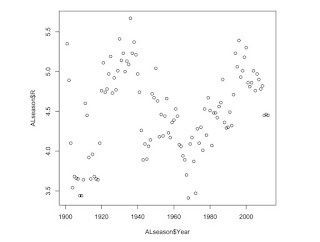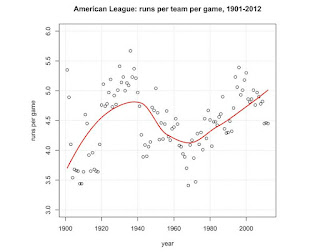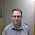July 14, 2012

Trends in AL run scoring (using R)

I have started to explore the functionality of R, the statistical and graphics programming language. And with what better data to play than that of Major League Baseball?

There have already been some good examples of using R to analyze baseball data. The most comprehensive is the on-going series at The Prince of Slides (Brian Mills, aka Millsy), cross-posted at the R-bloggers site. I am nowhere near that level, but explaining what I've done is a valuable exercise for me -- as Joseph Joubert said (no doubt in French) "To teach is to learn twice over."

So after some reading (I have found Paul Teetor's R Cookbook particularly helpful) and working through some examples I found on the web, I decided to plot some time series data, calculate a trend line, and then plot the points and trend line. I started with the American League data, from its origins in 1901 through to the All Star break of 2012.  For this, I relied on this handy table at Baseball Reference.

Step 1: load the data into the R workspace.  This required a bit of finessing in software outside R. Any text editor such as Notepad or TextPad would do the trick.  What I did was paste it into the text editor, tidied up the things listed below, and then saved the file with a .csv extension.

The source Baseball Reference table has some things that need to be remedied:
- There are bonus header rows throughout the table.  These need to be deleted.
- R uses the number sign (#) as an indicator for comments in the code; the Baseball Reference table has the number of batters in the league named “#Bat”. This field needs to be renamed before the data is read into R.

Here’s my line of R code to read the data:
This creates a table object "ALseason" that contains all the fields in the csv file.

A simple plot of the data points can be generated using the “plot” function:
plot(ALseason\$Year, ALseason\$R)
- This function uses the format plot(x, y).  In this case we want year on the x axis, and runs per game is on the y.
- R wants you to be precise – you have to indicate which table the variables are drawn from, so in this case the year variable needs to be specified as ALseasons\$Year. (As I’ve quickly discovered, there’s often more than one way to do something in R. And there is an alternative method for specifying variables in a table, but I’ll save that for another day.)A rudimentary plot of average runs scored in the American League, 1901-2012 All Star Break. (Click to enlarge.)

These data points are scattered across a 2 run range from lows below 3.5 to a maximum over 5.5, but at a glance the general trends are obvious and well-described in the histories of baseball. R gives on the tools to calculate a trend line around these points, using the LOESS method. (That's LOESS the regression method, not loess the aeolian sediment.)  I should note that Millsy used the LOESS method for plotting trends in Jeremy Guthrie's pitch speed.

The first thing to do is create a new object with the LOESS model, and a secondary one with the predicted values calculated by the model function.

ALRunScore.LO <- loess(ALseason\$R ~ ALseason\$Year)
ALRunScore.LO.predict <- predict(ALRunScore.LO)

Now to plot the points, with the predicted line superimposed.

# plot the data, add loess curve
ylim <- c(3,6)
plot(ALseason\$R ~ ALseason\$Year,
ylim = ylim,
main = "American League: runs per team per game, 1901-2012",
xlab = "year", ylab = "runs per game")
# plot loess predicted value line
lines(ALseason\$Year, ALRunScore.LO.predict,
lty="solid", col="red", lwd=2)
# chart tidying
grid()

This code also adds some features to the plot to tidy things up.
- The ylim object and function set the limits of the y axis from 3 to 6.  If you wanted the y axis to start at zero, your code specifying the variable ylim would be
ylim <- c(0,6)
- The main, xlab, and ylab add the main title and the x and y labels.
- The LOESS line is added through the lines function, using the (x, y) format described earlier.
- The lty, col, and lwd define the line type, colour, and weight.

Below is the output of the plotting code above.(click to enlarge)

Note that the LOESS used here is not particularly sensitive, and doesn't yet reflect the dramatic drop in run production that began in 2010 and has carried on to the present. The way to address this is to use the span specification, which makes it possible to adjust the degree of smoothing. This makes it more sensitive to the data points in the immediate vicinity rather than the longer-term trend. The smaller the span value, the more closely the line will follow the latest data points. (Here's a test of the control, using a sine curve.)

# create new object RunScore.LO for loess model, span=0.25 and 0.50
ALRunScore.LO.25 <- loess(ALseason\$R ~ ALseason\$Year, span=0.25)
ALRunScore.LO.25.predict <- predict(ALRunScore.LO.25)
#
ALRunScore.LO.5 <- loess(ALseason\$R ~ ALseason\$Year, span=0.5)
ALRunScore.LO.5.predict <- predict(ALRunScore.LO.5)
#

These additional predicted lines can be added to the plot code above. The code below also adds a legend to tell the lines apart.

# plot the data, add loess curve
ylim <- c(3,6)
plot(ALseason\$R ~ ALseason\$Year,
ylim = ylim,
main = "American League: runs per team per game, 1901-2012",
xlab = "year", ylab = "runs per game")
# loess predicted value line
lines(ALseason\$Year, ALRunScore.LO.predict, lty="solid", col="red", lwd=2)
lines(ALseason\$Year, ALRunScore.LO.25.predict, lty="dashed", col="blue", lwd=2)
lines(ALseason\$Year, ALRunScore.LO.5.predict, lty="dotdash", col="black", lwd=2)
# chart tidying
legend(1980, 3.5,
c("default", "span=0.25", "span=0.50"),
lty=c("solid", "dashed", "dotdash"),
col=c("red", "blue", "black"),
lwd=c(2, 2, 2))
grid()#(click to enlarge)

The two span lines change the predicted value quite dramatically -- most notably for recent years, where the default span value is about half a run higher than that of span=0.25.

Next time: adding the National League.

Data source:
- Baseball Reference

-30-

1 comment:

1.I used Excels "Data" "From Web" import wizard and the data came in perfectly. The only mod I had to do was remove the titles at the top of each page break.